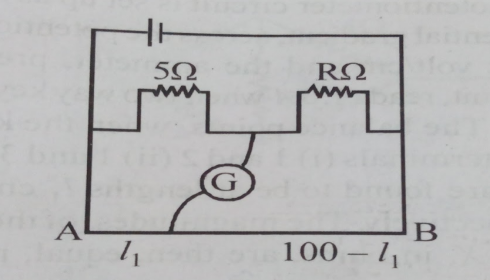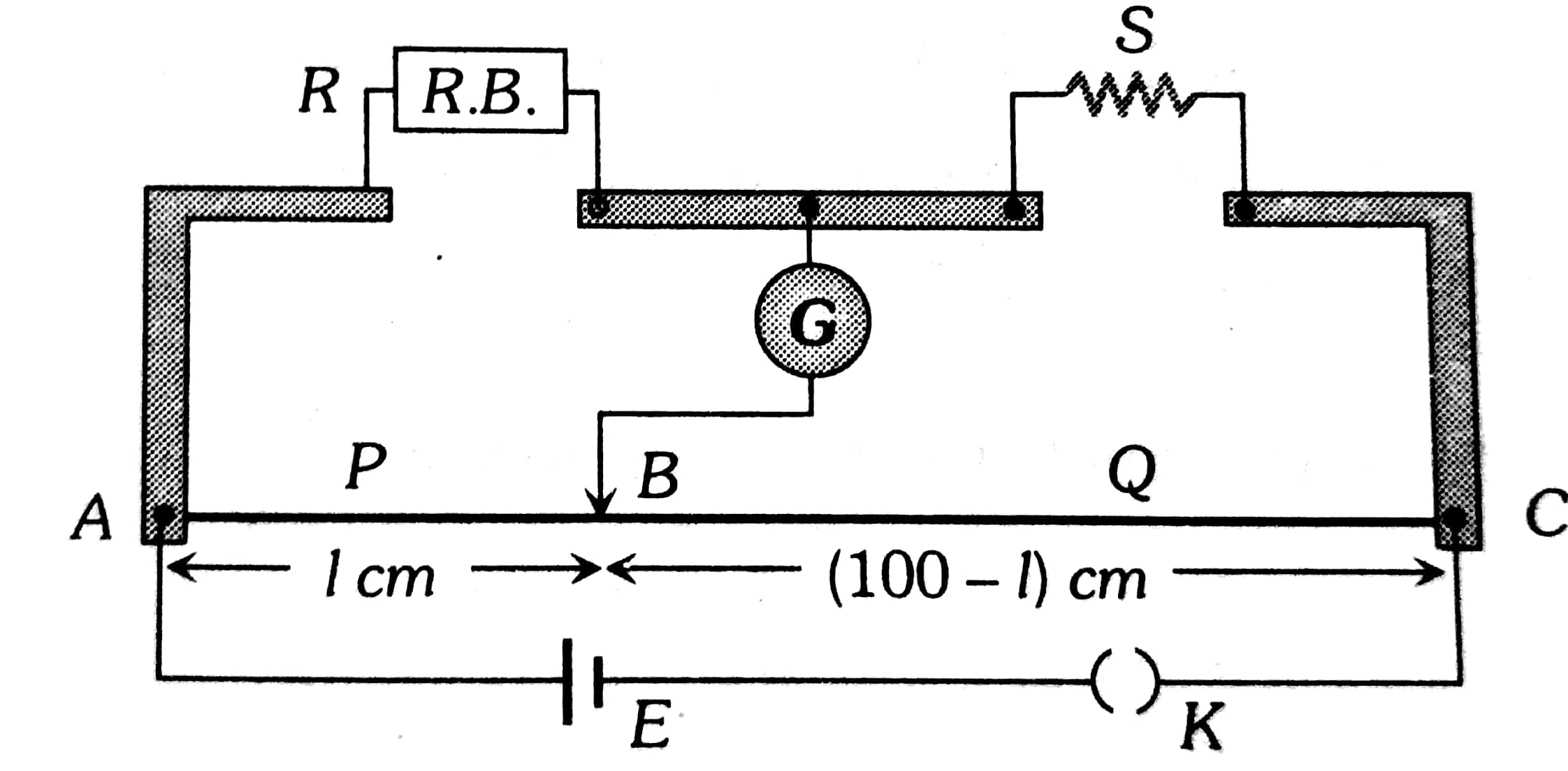# The resistance in the two arms of the meter bridge  are 5 and R, respectivilty. When the resistance R is shunted with an equal resistance, the new balance point is at . The resistance 'R' is:Option 1) 10 Option 2) 15 Option 3) 20 Option 4) 25

D Divya Saini

Meter bridge -

To find the resistance of a given wire using a meter bridge and hence determine the specific resistance of its materials

- whereinDivide  and

Or,

From equation

Option 1)

10

This option is incorrect.

Option 2)

15

This option is correct.

Option 3)

20

This option is incorrect.

Option 4)

25

This option is incorrect.

Exams
Articles
Questions